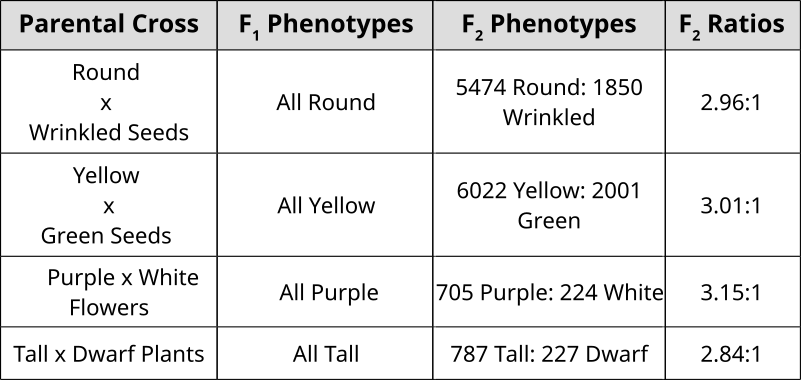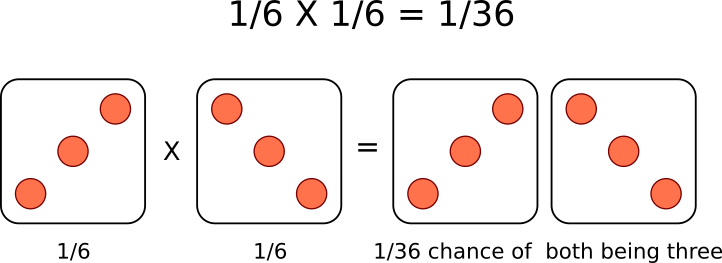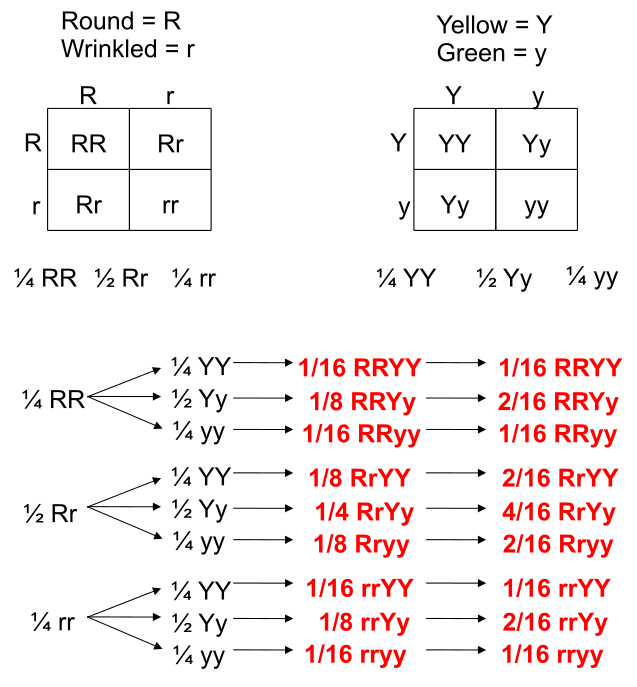# Probability and Chi-Square Analysis

## Mendel’s Observations## Probability: Past Punnett Squares

Punnett Squares are convenient for predicting the outcome of monhybrid or dihybrid crosses. The expectation of two heterozygous parents is 3:1 in a single trait cross or 9:3:3:1 in a two-trait cross. Performing a three or four trait cross becomes very messy. In these instances, it is better to follow the rules of probability. Probability is the chance that and event will occur expressed as a fraction or percentage. In the case of a monohybrid cross, 3:1 ratio means that there is a 3/4 (0.75) chance of the dominant phenotype with a 1/4 (0.25) chance of a recessive phenotype.A single die has a 1 in 6 chance of being a specific value. In this case, there is a 1/6 probability of rolling a 3. It is understood that rolling a second die simultaneously is not influenced by the first and is therefore independent. This second die also has a 1/6  chance of being a 3.

We can understand these rules of probability by applying them to the dihybrid cross and realizing we come to the same outcome as the 2 monohybrid Punnett Squares as with the single dihybrid Punnett Square.This forked line method of calculating probability of offspring with various genotypes and phenotypes can be scaled and applied to more characteristics.